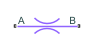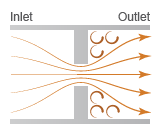# Local Restriction (MA)

Restriction in flow area in moist air network

•Libraries:
Simscape / Foundation Library / Moist Air / Elements

## Description

The Local Restriction (MA) block models the pressure drop due to a localized reduction in flow area, such as a valve or an orifice, in a moist air network. Choking occurs when the restriction reaches the sonic condition.

Ports A and B represent the restriction inlet and outlet. The input physical signal at port AR specifies the restriction area. Alternatively, you can specify a fixed restriction area as a block parameter.

The block icon changes depending on the value of the Restriction type parameter.

Restriction TypeBlock Icon

`Variable``Fixed`The restriction is adiabatic. It does not exchange heat with the environment.

The restriction consists of a contraction followed by a sudden expansion in flow area. The moist air accelerates during the contraction, causing the pressure to drop. The moist air separates from the wall during the sudden expansion, causing the pressure to recover only partially due to the loss of momentum.

Local Restriction SchematicCaution

Moist air flow through this block can choke. If a Mass Flow Rate Source (MA) block or a Controlled Mass Flow Rate Source (MA) block connected to the Local Restriction (MA) block specifies a greater mass flow rate than the possible choked mass flow rate, the simulation generates an error. For more information, see Choked Flow.

The block equations use these symbols.

 $\stackrel{˙}{m}$ Mass flow rate Φ Energy flow rate p Pressure ρ Density R Specific gas constant S Cross-sectional area Cd Discharge coefficient h Specific enthalpy cp Specific heat at constant pressure T Temperature

Subscripts `a`, `w`, and `g` indicate the properties of dry air, water vapor, and trace gas, respectively. Subscripts `lam` and `tur` indicate the laminar and turbulent regime, respectively. Subscripts `A` and `B` indicate the appropriate port. Subscript `R` indicates the restriction.

Mass balance:

`$\begin{array}{l}{\stackrel{˙}{m}}_{A}+{\stackrel{˙}{m}}_{B}=0\\ {\stackrel{˙}{m}}_{wA}+{\stackrel{˙}{m}}_{wB}=0\\ {\stackrel{˙}{m}}_{gA}+{\stackrel{˙}{m}}_{gB}=0\end{array}$`

Energy balance:

`${\Phi }_{A}+{\Phi }_{B}=0$`

When the flow is not choked, the mixture mass flow rate (positive from port A to port B) in the turbulent regime is

`$\begin{array}{l}{\stackrel{˙}{m}}_{tur}={C}_{d}{S}_{R}\left({p}_{A}-{p}_{B}\right)\sqrt{\frac{2{\rho }_{R}}{|{p}_{A}-{p}_{B}|{K}_{tur}}}\\ {K}_{tur}=\left(1+\frac{{S}_{R}}{S}\right)\left(1-\frac{{\rho }_{R}}{{\rho }_{in}}\frac{{S}_{R}}{S}\right)-2\frac{{S}_{R}}{S}\left(1-\frac{{\rho }_{R}}{{\rho }_{out}}\frac{{S}_{R}}{S}\right)\end{array}$`

Subscripts `in` and `out` indicate the inlet and outlet, respectively. If pApB, the inlet is port A and the outlet is port B; otherwise, they are reversed. The cross-sectional area S is assumed to be equal at ports A and B. SR is the area at the restriction.

The mixture mass flow rate equation is derived by combining the equations from two control volume analyses:

• Momentum balance for flow area contraction from the inlet to the restriction

• Momentum balance for sudden flow area expansion from the restriction to the outlet

In the analysis for the flow area contraction, pressure pin acts on the area at the inlet, S, and pressure pR acts on the area at the restriction, SR. The pressure acting on the area outside the restriction, SSR, is assumed to be (pinS + pRSR)/(S + SR).

In the analysis for the flow area expansion, the pressure acting on both the area at the restriction, SR, and the area outside the restriction, SSR, is assumed to be pR, because of flow separation from the restriction. The pressure acting on the area at the outlet , S, is equal to pout.

The mixture mass flow rate (positive from port A to port B) in the laminar regime is linearized with respect to the pressure difference:

`${\stackrel{˙}{m}}_{lam}={C}_{d}{S}_{R}\left({p}_{A}-{p}_{B}\right)\sqrt{\frac{2{\rho }_{R}}{\Delta {p}_{threshold}{\left(1-\frac{{S}_{R}}{S}\right)}^{2}}}$`

where the threshold for transition between the laminar and turbulent regime is defined based on the laminar flow pressure ratio, Blam, as

`$\Delta {p}_{threshold}=\left(\frac{{p}_{A}+{p}_{B}}{2}\right)\left(1-{B}_{lam}\right)$`

When $|{p}_{A}-{p}_{B}|\ge \Delta {p}_{threshold}$, the flow is assumed to be turbulent and therefore ${\stackrel{˙}{m}}_{unchoked}={\stackrel{˙}{m}}_{tur}$.

When $|{p}_{A}-{p}_{B}|<\Delta {p}_{threshold}$, ${\stackrel{˙}{m}}_{unchoked}$ smoothly transitions to ${\stackrel{˙}{m}}_{lam}$.

When the flow is choked, the velocity at the restriction is equal to the speed of sound and cannot increase any further. Assuming the flow is choked, the mixture mass flow rate is

`${\stackrel{˙}{m}}_{choked}={C}_{d}{S}_{R}{p}_{R}\sqrt{\frac{{\gamma }_{R}}{R{T}_{R}}}$`

where ${\gamma }_{R}={c}_{pR}/\left({c}_{pR}-R\right)$. Therefore, the actual mixture mass flow rate is equal to ${\stackrel{˙}{m}}_{unchoked}$, but is limited in magnitude by ${\stackrel{˙}{m}}_{choked}$:

The expression for the pressure at the restriction is obtained by considering the momentum balance for flow area contraction from the inlet to the restriction only.

`${p}_{R}={p}_{in}-\frac{1}{2{\rho }_{R}}{\left(\frac{{\stackrel{˙}{m}}_{A}}{{C}_{d}{S}_{R}}\right)}^{2}\left(1+\frac{{S}_{R}}{S}\right)\left(1-\frac{{\rho }_{R}}{{\rho }_{in}}\frac{{S}_{R}}{S}\right)$`

The local restriction is assumed adiabatic, so the mixture specific total enthalpies are equal. Therefore, the changes in mixture specific enthalpies are:

`$\begin{array}{l}{h}_{A}-{h}_{R}=\left(\frac{1}{{\rho }_{R}^{2}{S}_{R}^{2}}-\frac{1}{{\rho }_{A}^{2}{S}^{2}}\right)\frac{{\stackrel{˙}{m}}_{A}^{2}}{2{C}_{D}^{2}}\\ {h}_{B}-{h}_{R}=\left(\frac{1}{{\rho }_{R}^{2}{S}_{R}^{2}}-\frac{1}{{\rho }_{B}^{2}{S}^{2}}\right)\frac{{\stackrel{˙}{m}}_{B}^{2}}{2{C}_{D}^{2}}\end{array}$`

### Assumptions and Limitations

• The restriction is adiabatic. It does not exchange heat with the environment.

• This block does not model supersonic flow.

## Ports

### Input

expand all

Input physical signal that controls the air flow restriction area. The signal saturates when its value is outside the minimum and maximum restriction area limits, specified by the block parameters.

#### Dependencies

This port is visible only if you set the Restriction type parameter to `Variable`.

### Conserving

expand all

Moist air conserving port associated with the inlet or outlet of the local restriction. This block has no intrinsic directionality.

Moist air conserving port associated with the inlet or outlet of the local restriction. This block has no intrinsic directionality.

## Parameters

expand all

Select whether the restriction area can change during simulation:

• `Variable` — The input physical signal at port AR specifies the restriction area, which can vary during simulation. The Minimum restriction area and Maximum restriction area parameters specify the lower and upper bounds for the restriction area.

• `Fixed` — The restriction area, specified by the Restriction area block parameter value, remains constant during simulation. Port AR is hidden.

Lower bound for the restriction cross-sectional area. You can use this parameter to represent the leakage area. The input signal AR saturates at this value to prevent the restriction area from decreasing any further.

#### Dependencies

Enabled when the Restriction type parameter is set to `Variable`.

Upper bound for the restriction cross-sectional area. The input signal AR saturates at this value to prevent the restriction area from increasing any further.

#### Dependencies

Enabled when the Restriction type parameter is set to `Variable`.

Area normal to flow path at the restriction.

#### Dependencies

Enabled when the Restriction type parameter is set to `Fixed`.

Area normal to flow path at ports A and B. This area is assumed to be the same for the two ports.

Ratio of actual mass flow rate to the theoretical mass flow rate through the restriction. The discharge coefficient is an empirical parameter that accounts for nonideal effects.

Pressure ratio at which the moist air flow transitions between laminar and turbulent regimes. The pressure loss is linear with respect to mass flow rate in the laminar regime and quadratic with respect to mass flow rate in the turbulent regime.

## Version History

Introduced in R2018a